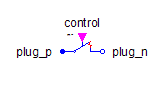AIM Slip Ring - MapleSim Help

Fundamental Wave AIM Slip Ring

Asynchronous Induction Machine with Slip Ring RotorDescription

The Fundamental Wave AIM Slip Ring (or AIM Slip Ring) component models an asynchronous induction machine with a slip ring rotor. Resistances and stray inductances of the machine always refer to either stator or rotor. The symmetry of the stator and rotor is assumed. The number of stator and rotor phases may be different. The following losses are modeled:

 • heat losses in the temperature dependent stator winding resistances
 • heat losses in the temperature dependent rotor winding resistances
 • friction losses
 • stator and rotor core losses (only eddy current losses, no hysteresis losses)Connections

 Name Description Modelica ID $\mathrm{powerBalance}$ Power balance powerBalance $\mathrm{flange}$ Shaft flange $\mathrm{support}$ Support at which the reaction torque is acting support ${\mathrm{plug}}_{\mathrm{sp}}$ Positive plug of stator plug_sp ${\mathrm{plug}}_{\mathrm{sn}}$ Negative plug of stator plug_sn $\mathrm{thermalPort}$ Thermal port of induction machines thermalPort ${\mathrm{plug}}_{\mathrm{rn}}$ Negative plug of rotor plug_rn ${\mathrm{plug}}_{\mathrm{rp}}$ Positive plug of rotor plug_rpParametersGeneral Parameters

 Name Default Units Description Modelica ID $\mathrm{mr}$ $m$ Number of rotor phases mr $m$ $3$ Number of stator phases m ${J}_{r}$ $\mathrm{kg}{m}^{2}$ Rotor moment of inertia Jr Use Support Flange $\mathrm{false}$ True (checked) means enable stator support flange useSupport ${J}_{s}$ $\mathrm{kg}{m}^{2}$ Stator moment of inertia Js Use Thermal Port $\mathrm{false}$ True (checked) means thermal port is enabled useThermalPort $p$ Number of pole pairs (Integer) p ${f}_{s,\mathrm{nom}}$ $\mathrm{Hz}$ Nominal frequency fsNominal ${T}_{s,\mathrm{oper}}$ $K$ Operational temperature of stator resistance TsOperational Effective Stator Turns $1$ $1$ Effective number of stator turns effectiveStatorTurns ${T}_{r,\mathrm{oper}}$ $K$ Operational temperature of rotor resistance TrOperational Use Turns Ratio $\mathrm{true}$ Use TurnsRatio or calculate from locked-rotor voltage? useTurnsRatio $\mathrm{Turns Ratio}$ $1$ $1$ Effective number of stator turns / effective number of rotor turns TurnsRatio ${V}_{s,\mathrm{nom}}$ $100$ $V$ Nominal stator voltage per phase VsNominal ${V}_{r,\mathrm{locked}}$ $V$ Locked rotor voltage per phase VrLockedRotorLosses Parameters

 Name Default Units Description Modelica ID Friction Parameters Friction loss parameter record frictionParameters Stator Core Parameters Stator core loss parameter record; all parameters refer to stator side statorCoreParameters Stray Load Parameters Stray load loss parameter record strayLoadParameters Rotor Core Parameters Rotor core loss parameter record, all quantities refer to rotor side rotorCoreParametersNominal Resistances And Inductances Parameters

 Name Default Units Description Modelica ID ${R}_{s}$ $0.03$ $\mathrm{\Omega }$ Warm stator resistance per phase Rs ${T}_{s,\mathrm{ref}}$ $293.15$ $K$ Reference temperature of stator resistance TsRef ${\mathrm{\alpha }}_{s}$ $0$ $\frac{1}{K}$ Temperature coefficient of stator resistance at 20 degC alpha20s ${L}_{s\sigma }$  $H$ Stator stray inductance per phase Lssigma ${L}_{s,0}$ ${L}_{s\sigma }$ $H$ Stator zero inductance Lszero ${L}_{m}$  $H$ Main field inductance Lm ${L}_{r\sigma }$  $H$ Rotor stray inductance per phase Lrsigma ${L}_{r,0}$ ${L}_{r\sigma }$ $H$ Rotor zero inductance w.r.t. rotor side Lrzero ${R}_{r}$ $0.04$ $\mathrm{\Omega }$ Warm rotor resistance per phase Rr ${T}_{r,\mathrm{ref}}$ $293.15$ $K$ Reference temperature of rotor resistance TrRef ${\mathrm{\alpha }}_{r}$ $0$ $\frac{1}{K}$ Temperature coefficient of rotor resistance at 20 degC alpha20r

 $\frac{3\left(1-\sqrt{\frac{14}{15}}\right)}{2\mathrm{\pi }{f}_{s,\mathrm{nom}}}$

 $\frac{3\sqrt{\frac{14}{15}}}{2\mathrm{\pi }{f}_{s,\mathrm{nom}}}$Modelica Standard Library The component described in this topic is from the Modelica Standard Library. To view the original documentation, which includes author and copyright information, click here.